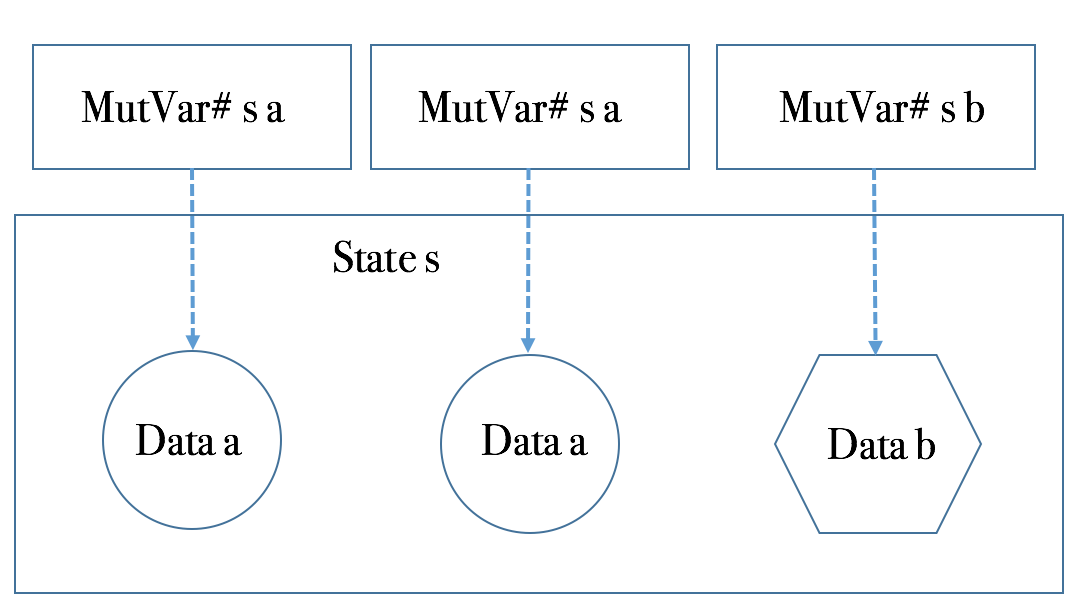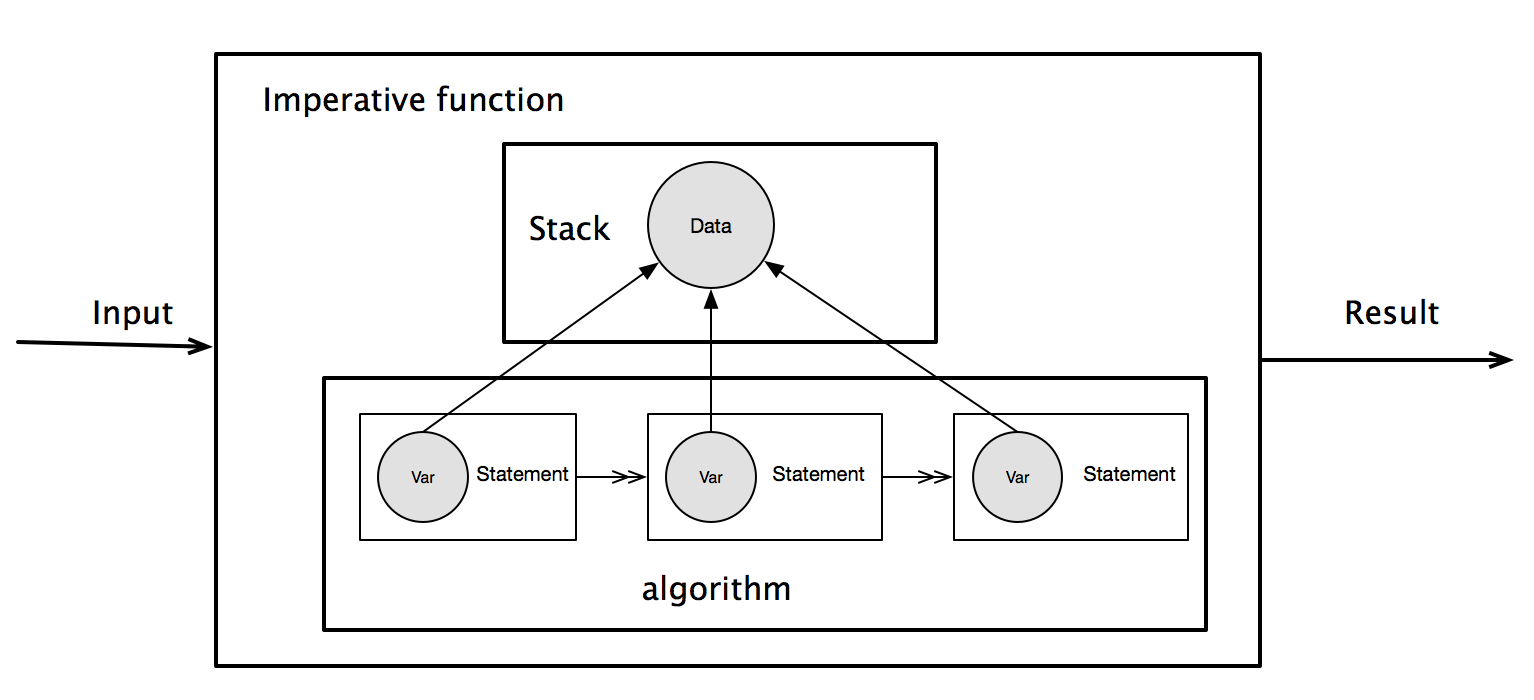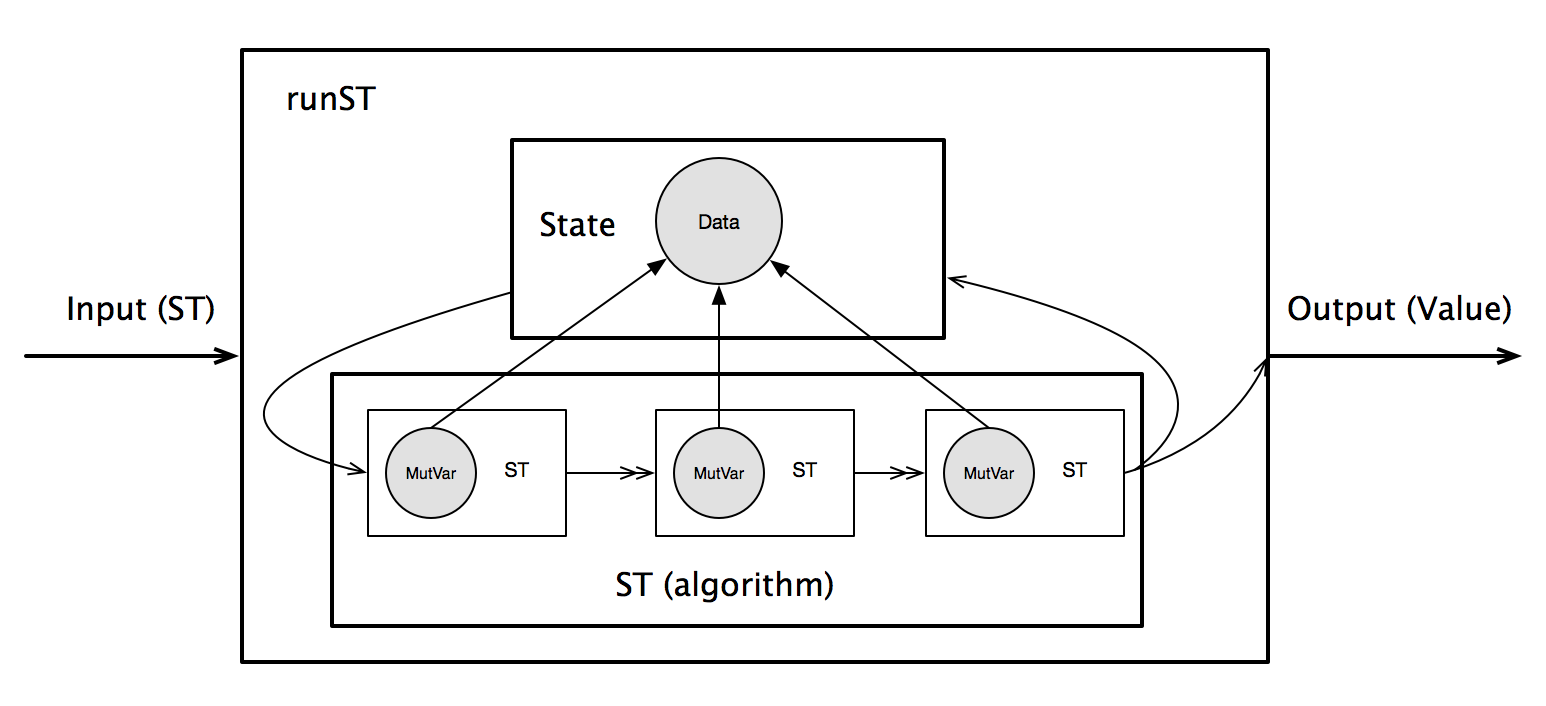# Immutability：The Dark Side

FPer对OO批评最多的是：由于OO允许在运行时修改状态，从而无法做到引用透明(Referiential Transparency)。换句话说：对于同一个函数，使用相同的输入参数，每次调用返回的结果却不相同。比如：

struct Foo
{
int inc(int b)
{
a += b;
return a;
}

private:
int a = 1;
};

void f()
{
Foo foo;

std::cout << foo.inc(2) << std::endl; // 3
std::cout << foo.inc(2) << std::endl; // 5
}

struct Foo
{
Foo(int a) : a(a) {}

Foo inc(int b) const
{
return a + b;
}

private:
int a = 1;
}; 

Do not communicate by sharing memory; instead, share memory by communicating.

## 性能永远都是一个问题

quicksort []     = []
quicksort (p:xs) = (quicksort lesser) ++ [p] ++ (quicksort greater)
where
lesser  = filter (< p) xs
greater = filter (>= p) xs

void qsort(int a[], int lo, int hi)
{
int h, l, p, t;

if (lo < hi) {
l = lo;
h = hi;
p = a[hi];

do {
while ((l < h) && (a[l] <= p))
l = l+1;
while ((h > l) && (a[h] >= p))
h = h-1;
if (l < h) {
t = a[l];
a[l] = a[h];
a[h] = t;
}
} while (l < h);

a[hi] = a[l];
a[l] = p;

qsort( a, lo, l-1 );
qsort( a, l+1, hi );
}
}

-- A MutVar# behaves like a single-element mutable array.
data MutVar# s a

-- Create MutVar# with specified initial value in specified state thread.
newMutVar# :: a -> State# s -> (# State# s, MutVar# s a #)

-- Read contents of MutVar#. Result is not yet evaluated.
readMutVar# :: MutVar# s a -> State# s -> (# State# s, a #)

-- Write contents of MutVar#.
writeMutVar# :: MutVar# s a -> a -> State# s -> State# s

data State# s

MutVar#是一个类型构造器，有两个参数：a是要存储的数据类型。而s则代表是状态。从逻辑上，MutVar#自身是一个引用，其引用的数据类型为a，而空间则从s所管理的存储上分配。如下图所示。calc' :: (Num a) => State# s -> a -> a
calc' initS initV =
let (# s1, ref #) = newMutVar# initV initS

(# s2, v1 #) = readMutVar# ref s1
s3 = writeMutVar# ref (v1*10)  s2
(# s4, v2 #) = readMutVar# ref s3
s5 = writeMutVar# ref (v2+20)  s4

(# _finalS, finalV #) = readMutVar# ref s5
in finalV

1. 在初始状态分配一个内容为初始值的数据，然后得到一个引用，以及被修改的状态；
2. 不断通过引用，读写数据内容；注意，中间状态也在不断改变和传递；
3. 最后，丢弃最终状态，从引用里读出数据返回。

newtype ST s a = ST (STRep s a)
type STRep s a = State# s -> (# State# s, a #)

return x = ST (\ s -> (# s, x #))

(ST m) >>= k
= ST $\s -> case (m s) of { (# new_s, r #) -> case (k r) of { ST k2 -> (k2 new_s) }} 为了方便于Monad内部的代码编写，以及类型系统的约束，再提供如下辅助函数：  data STRef s a = STRef (MutVar# s a) newSTRef :: a -> ST s (STRef s a) newSTRef init = ST$ \s1# ->
case newMutVar# init s1#            of { (# s2#, var# #) ->
(# s2#, STRef var# #) }

readSTRef :: STRef s a -> ST s a
readSTRef (STRef var#) = ST $\s1# -> readMutVar# var# s1# writeSTRef :: STRef s a -> a -> ST s () writeSTRef (STRef var#) val = ST$ \s1# ->
case writeMutVar# var# val s1#      of { s2# ->
(# s2#, () #) }

modifySTRef :: STRef s a -> (a -> a) -> ST s ()
modifySTRef ref f = writeSTRef ref . f =<< readSTRef ref   

calc' ::  Int -> ST s Int
calc' initV = do
ref <- newSTRef initV
writeSTRef $v1 * 10 v2 <- readSTRef ref writeSTRef$ v2 + 20
readSTRef ref

runST :: (forall s. ST s a) -> a
runST st = runSTRep (case st of { ST st_rep -> st_rep })

runSTRep :: (forall s. STRep s a) -> a
runSTRep st_rep = case st_rep realWorld# of
(# _, r #) -> r

runST里，一个具体的状态实例作为参数传给了State Transformer。而这个实例，则是GHC编译器提供的readWorld#，其类型为GHC内置的ReadWorld#

calc :: Int -> Int
calc initValue = runST $calc' initValue ## 对命令式的模仿 runST是一个重要的边界：它创建了一个初始状态，传递给State Transformer，然后得到一个最终状态，以及计算的结果。最后，抛弃掉最终状态，仅保留计算结果。 如果我们把这一系列的机制和命令式语言对应起来，可以看出，这完全是对命令式语言局部变量处理的模拟。 对于命令式语言，所有的局部变量都是在线程的栈上分配，从而修改了Stack的状态。随后所有的读写操作也都在栈上进行，不断修改Stack的状态。在一个函数处理结束后，在栈上分配的局部变量都会自动释放，关心的数据则当作函数的返回值返回。如下图所示。而在ST Monad里，runST对应的是命令式语言的函数，而Stack对应的则是State：在计算开始时，提供一个State，计算在State上分配数据，存取State的状态，等计算结束后，State的最终状态被抛弃，返回计算结果。如下图所示。这样的模仿，相对于命令式语言的局部变量可变性，并未得到任何额外的好处。但很明显，命令式语言在这类问题上的处理要简洁的多。同时也说明了，允许局部变量的可修改性，对于引用透明和线程安全没有任何影响，只会带来简洁和性能。 ## Rank 2 Type 我们设想一下，在命令式语言里，在一个函数调用结束后，如果其在栈里的分配的数据不释放，可以被随后的函数访问，那么那些数据就与全局变量无异了。我们都知道，全局变量是违背Shared Nothing原则的。 通过上述对照，我们知道State是对Stack的模仿。在runST结束后，State也必须像Stack一样被释放，避免被其它计算继续使用，这才能保证线程安全。 可是，如果我们写下这样的代码： f1 :: STRef s a -> ST s a f1 ref = do v <- readSTRef ref writeSTRef$ v * 10

let ref = runST $newSTRef 10 v1 = runST$ readSTRef ref
v2  = runST $f1 ref in ... 这就造成了ref在多个runST之间传递。对于惰性求值的语言而言，runST的求值顺序是不确定的，从而导致v1v2计算结果的不确定。这就像多线程程序共享同一份数据，却没有任何措施来保证对共享数据访问的序列化一样，是一个严重的问题。 不过，ST设计者通过引入Rank 2 Types解决了这个问题。Rank 2 Types不属于Hindley-Milner类型系统的范畴。也不在haskell 98里。但这对于很多设计却又是比不可少的。 在Rank 1 Type的一个函数里，每个类型变量，只能被绑定为一个类型。这就导致了，如果一个高阶函数的某个参数是一个参数化多态（Parametric Polymorphism）函数，那么在这个高阶函数里，无论那个参数化多态函数被调用多少次，其类型变量所绑定的类型都必须是一致的。比如下面的代码就无法通过编译： g :: (a -> a) -> (Bool, Char) g f = (f True, f 'c') 因为，f在同一个函数g的上下文里，两次调用里，其类型变量a被绑定的类型是不同的：一个是Bool，一个是Char 这明显是一个给程序员带来麻烦的问题。因而，GHC给出了一个扩展，叫Rank2Types。它允许程序员通过将上面例子中函数g的类型修改为： g :: (forall a. a -> a) -> (Bool, Char) 某种程度上，你可以将Rank 2 Types理解为C++的Template Template Parameter技术，比如： template <typename T, typename G, template <typename E> class Container > struct Foo { Container<T> tContainer; Container<G> gContainer; }; 下面我们来看看为何Rank 2 Type可以解决runST状态泄漏的问题。我们先来推导一下表达式runST$ newSTRef 10的类型：

runST :: (forall s. ST s a) -> a
newSTRef 10 :: forall s. ST s (STRef s Int)
[STRef s Int ~ a]

runST $newSTRef 10 :: (forall s. ST s (STRef s Int)) -> STRef s Int 其中，s已经出现在$$\forall s$$的限定范围之外，因而编译器将不会通过编译。 但是，如果runST的类型为forall s. ST s a -> a，上述类型则变为： runST$ newSTRef 10 :: forall s. ST s (STRef s Int) -> STRef s Int

$$\frac{ { \ldots, s=State\ RealWorld,\ ref=STRef\ \ s\ \ Int } }{b}$$

$\frac{{\dots, s=State#\ \ RealWorld#,\ ref=STRef\ \ s\ \ Int } \vdash readVar\ \ ref::ST\ \ s\ \ Int}{\Gamma \vdash readVar\ \ ref::ST\ \ (State#\ \ RealWorld#)\ \ Int}$

## 模仿C语言算法

import Control.Monad (when)
import Data.Array.ST
import Data.Array.IArray
import Data.Array.MArray

qsort :: (IArray a e, Ix i, Enum i, Ord e) => a i e -> a i e
qsort arr = processArray quickSort arr

processArray :: (IArray a e, IArray b e, Ix i)
=> (forall s. (STArray s) i e -> ST s ()) -> a i e -> b i e
processArray f (arr :: a i e) = runST $do arr' <- thaw arr :: ST s (STArray s i e) f arr' unsafeFreeze arr' quickSort :: (MArray a e m, Ix i, Enum i, Ord e) => a i e -> m () quickSort arr = qsort' =<< getBounds arr where qsort' (lo, hi) | lo >= hi = return () | otherwise = do p <- readArray arr hi l <- mainLoop p lo hi swap l hi qsort' (lo, pred l) qsort' (succ l, hi) mainLoop p l h | l >= h = return l | otherwise = do l' <- doTil (\l' b -> l' < h && b <= p) succ l h' <- doTil (\h' b -> h' > l' && b >= p) pred h when (l' < h') &\$& do
swap l' h'
mainLoop p l' h'

doTil p op ix = do
if p ix b then doTil p op (op ix) else return ix

swap xi yi = do
readArray arr yi >>= writeArray arr xi
writeArray arr yi x

The program below is working very very slowly. It's probably slowsort... :o)

import Data.Array.Base (unsafeRead, unsafeWrite)
import Data.Array.ST

myqsort :: STUArray s Int Int -> Int -> Int -> ST s ()
myqsort a lo hi
| lo < hi   = do
let lscan p h i
| i < h = do
if p < v then return i else lscan p h (i+1)
| otherwise = return i
rscan p l i
| l < i = do
if v < p then return i else rscan p l (i-1)
| otherwise = return i
swap i j = do
unsafeRead a j >>= unsafeWrite a i
unsafeWrite a j v
sloop p l h
| l < h = do
l1 <- lscan p h l
h1 <- rscan p l1 h
if (l1 < h1) then (swap l1 h1 >> sloop p l1 h1) else return l1
| otherwise = return l
i <- sloop piv lo hi
swap i hi
myqsort a lo (i-1)
myqsort a (i+1) hi
| otherwise = return ()

... reports that this version runs within 2x of the C version.

## 结论

「软件匠艺社区」旨在传播匠艺精神，通过分享好的「工作方式」和「习惯」以帮助程序员更加快乐高效地编程。